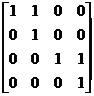§ 6   Rowdang canonical form of square matrix

1. Invariant subspace

Let L be a linear transformation of a real (or complex) linear space V , and S be a subspace of V, if , then S is an invariant subspace about L.Let be an invariant subspace of a linear transformation L of an n -dimensional linear space V , and V can use their direct sum: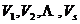The necessary and sufficient condition to represent is: the matrix A corresponding to the linear transformation L can be reduced to a block diagonal matrix under a certain basisThe order in the formula is equal to the dimension of .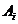Second, the standardization of the square matrix

The form of [ Ruodang block and Ruodang standard square matrix ]   isThe m -order square matrix of is called the Rodang block, where is an eigenvalue .The sub-matrix of a block matrix of a square matrix on the main diagonal is a block, and the rest of the sub-matrix are zero matrices, that is,( 1 )

Then it is called the Rowdang standard square matrix or the Rowdang standard form . Note that these are not necessarily different in different blocks .[ Standardization of Square Matrix ]

1 o In the case of different eigenvalues ​​If the eigenvalues ​​of a square matrix A are not equal, then A can be transformed into a diagonal matrix,

The elements on its main diagonal are these eigenvalues:2 o When the eigenvalues ​​are equal, any square matrix A can be transformed into its similar Rowdang standard form ( 1 ), whereis its eigenvalue and is the multiplicity of eigenvalues . If the order of the blocks is ignored, the canonical form of A is unique .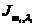It can be reduced to a diagonal matrix if and only if the order of all the blocks is equal to 1. This is the case of 1 o .As explained above, assuming that A is a square matrix, then a non-singular square matrix T can always be found , making the square matrix similar to A.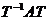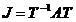3. Methods and steps of square matrix standardization

[ λ Matrix ]   Assuming that the elements of an n -order square matrix A are all complex coefficient polynomials of variable λ , it is called a λ matrix . The highest order r of a sub-form of a λ matrix that is not equal to zero is called the rank .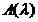[ Invariant factor and elementary factor ]  Let r be the rank, k is a positive integer , and the highest common factor of all k -order sub-formulas of is a polynomial, and the specified coefficient of the highest-order term is 1 ; in addition, it is specified that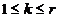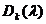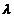say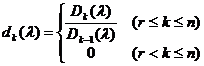invariant factor for .Decompose each into a first-order factor, we get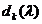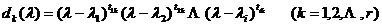Some of the exponents in the formula may be zero, and at that time , it is called an elementary factor of .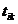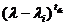[ Elementary Transformation · Matrix Equivalence ]   A finite combination of the following three transformations on the λ matrix is ​​called an elementary transformation .( i ) any two rows (columns) are interchanged;

( ii ) Multiply each element of any row (column) by the same λ polynomial and add it to the corresponding element of another row (column);

( iii ) Multiply any row (column) element by the same complex number not equal to zero .

It should be noted that, properly implementing ( ii ), ( iii ) both transformations can yield ( i ) .

If it can be obtained by a finite number of elementary transformations, it is said to be equivalent to .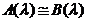After the λ matrix undergoes elementary transformation, its invariant factor and elementary factor remain unchanged .

[ Standard form of λ matrix ] Let the rank of the λ matrix be r and the invariant factor be , then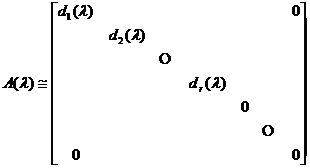Call the square matrix on the right the canonical form . It is determined by unique .Equivalent λ matrices have the same canonical form .

[ eigenmatrix ]   The eigenmatrix of square matrix A is a special λ matrix . So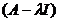1 o If the elementary factor is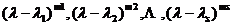which are not necessarily different from each other, then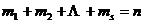and have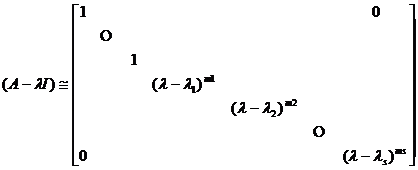2 o if the nth order λ matrixAmong them , then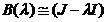where J is the standard form of A.

3 o If the elementary factor of the characteristic matrix of A isbut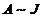If J is the standard form of A.

[ Steps of Square Matrix Standardization ] The steps   to convert the square matrix A into the standard form of A are as follows:

(1)  Use elementary transformation to convert it into a diagonal matrix, decompose the polynomial on the diagonal, and get all the elementary factors .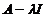(2)   Corresponding to each elementary factor , make a m -order Row-Dang block(3)   Combine all the Ruodang blocks to get the Ruodang canonical form of A.

Example 1 to   find a square matrix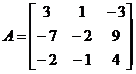The Jodang standard form of .

untie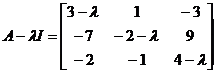It is easy to find that its invariant factors are 1 , 1 , , so the elementary factors are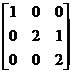Example 2   Find a square matrix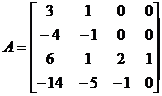The Jodang standard form of .

untie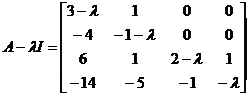After elementary transformation, it can be transformed into a diagonal matrix of the following formSo the elementary factor is , , and the corresponding if-dang block is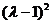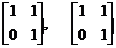Therefore , the standard form of A 's jodang is